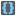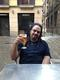• # Stuck

## Question related to missionBrackets

I can't figure out what is wrong here:

def checkio(expression):

```brackets = '{}[]()'
li = [x for x in expression if x in brackets]
pos = 0

while len(li) > 1:

if li[pos] == '{' and li[pos+1] == '}':
li.remove(li[pos])
li.remove(li[pos])
pos = 0
elif li[pos] == '[' and li[pos+1] == ']':
li.remove(li[pos])
li.remove(li[pos])
pos = 0
elif li[pos] == '(' and li[pos+1] == ')':
li.remove(li[pos])
li.remove(li[pos])
pos = 0
elif li[pos] == '}' or li[pos] == ']' or li[pos] == ')':
break
else:
pos += 1

if len(li) == 0:
return True
else:
return False
```

My logic is clearly to compare each bracket, remove them if they match, and then start over from the beginning. If no match is found by the time we get to closing brackets, no match will be found and we can break and return false. I get all the way to the really long example (3.5) and it returns False. Any help would be appreciated.9